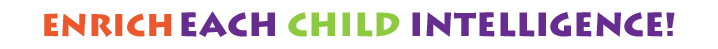Welcome to Our nursery!

•# Explore Our Events9 AM 1 PM function makeTimer4() { var e = new Date("2/11/2021"), e = Date.parse(e) / 1e3, a = new Date, a = Date.parse(a) / 1e3, t = e - a, o = Math.floor(t / 86400), i = Math.floor((t - 86400 * o) / 3600), s = Math.floor((t - 86400 * o - 3600 * i) / 60), n = Math.floor(t - 86400 * o - 3600 * i - 60 * s); i < "10" && (i = "0" + i), s < "10" && (s = "0" + s), n < "10" && (n = "0" + n), \$("#days4").html(o + "
"), \$("#hours4").html(i + "
"), \$("#minutes4").html(s + "
"), \$("#seconds4").html(n + "
") } setInterval(function() { makeTimer4() }, 1e3); Days Hours Minutes SecondsAl Shamkha branch Opening 7:00AM to 5:00PM 24.357916, 54.699211 Submitting the files successfully ADEK Inspection NOC The nursery is open Now your child can received the education and the support they have waited for. Limited seats are available call now and book a tour. IQ Kids Nursery Shamkha branch 056 752 2242 02 641 1412 function makeTimer3() { var e = new Date("11/30/2020"), e = Date.parse(e) / 1e3, a = new Date, a = Date.parse(a) / 1e3, t = e - a, o = Math.floor(t / 86400), i = Math.floor((t - 86400 * o) / 3600), s = Math.floor((t - 86400 * o - 3600 * i) / 60), n = Math.floor(t - 86400 * o - 3600 * i - 60 * s); i < "10" && (i = "0" + i), s < "10" && (s = "0" + s), n < "10" && (n = "0" + n), \$("#days3").html(o + "
"), \$("#hours3").html(i + "
"), \$("#minutes3").html(s + "
"), \$("#seconds3").html(n + "
") } setInterval(function() { makeTimer3() }, 1e3); Days Hours Minutes Seconds